##### Linear Algebra For Dummies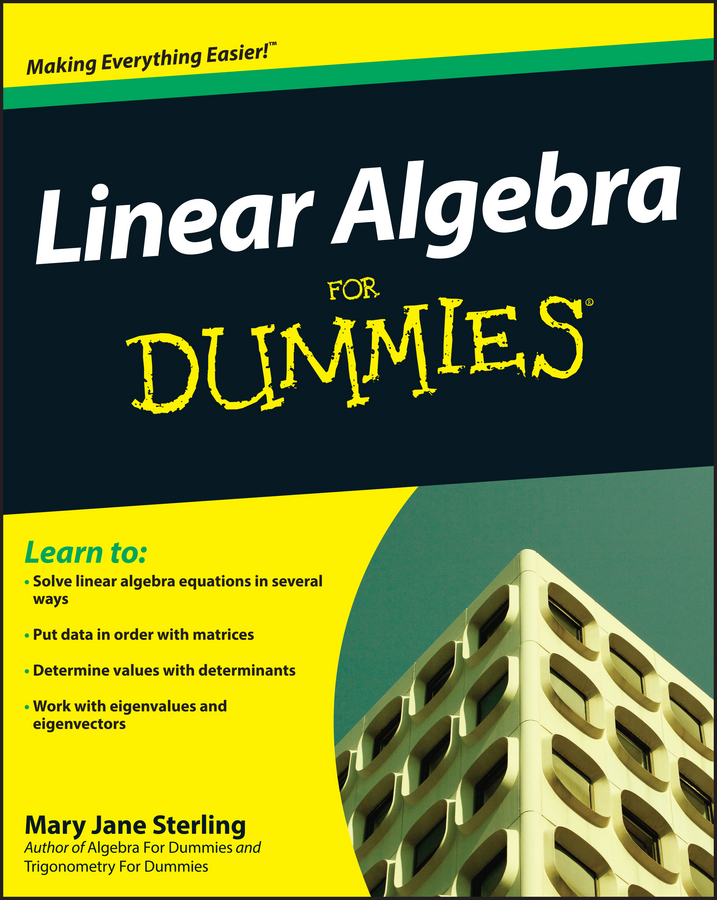To factor the difference of two perfect cubes, remember this rule: the difference of two perfect cubes equals the difference of their cube roots multiplied by the sum of their squares and the product of their cube roots. The binomial expression looks like this: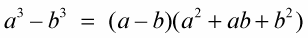The results of factoring the difference of perfect cubes are

• A binomial factor (ab) made up of the two cube roots of the perfect cubes separated by a minus sign.

If the cube isn’t there, and the number is smaller than the largest cube on the list, then the number isn’t a perfect cube. For bigger numbers, use a scientific calculator and the cube root button.

• A trinomial factor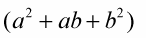• made up of the squares of the two cube roots added to the product of the cube roots in the middle.

The most well-known perfect cubes are those whose roots are integers, not decimals. Becoming familiar with and recognizing these cubes in an algebra problem can save time and improve accuracy. Variable cubes are relatively easy to spot because their exponents are always divisible by 3. When a number is cubed and multiplied out, you can’t always tell it’s a cube unless you memorize a list of the cubes.

Example 1: Factor the difference between the cubes, 216 – 125.

1. Use the difference of cubes rule to find the variables.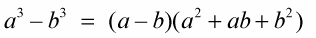In this case,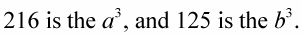The cube root of 216 is 6, and the cube root of 125 is 5; so 6 is the a, and 5 is the b.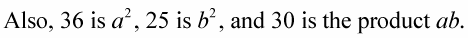2. Substitute the values into the equation.

216 – 125 = (6 – 5)(36 + 30 + 25).

3. Check to see if the equation is true.

The difference between 216 and 125 is 91.

Also, 6 – 5 = 1, and 36 + 30 + 25 = 91; so (1)(91) = 91.

Whether the expression is the difference of the two cubes or the factored form, the answer comes out the same.

Example 2: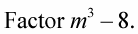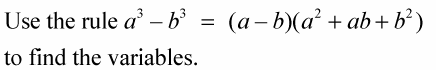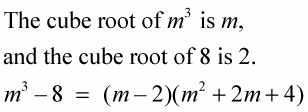Notice that the sign between the m and the 2 is the same as the sign between the cubes.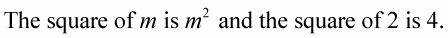The product of the two cube roots is 2m, and the signs in the trinomial are all positive.